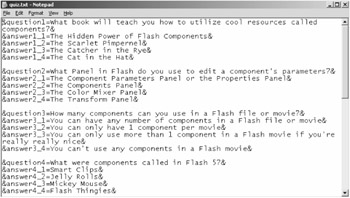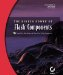# Customizing the Images

Of course, the main content for any quiz game is the set of questions. The questions are in the quiz.txt file. The questions can be in any text file, but they do need to be set up in a specific format. Let's look at the quiz.txt file. You can open quiz.txt in any text editor, such as Notepad (see Figure 9.7).Figure 9.7: The quiz.txt file conforms to a specific structure in order to work properly with the Quiz component in Flash.

Let's look at the first line in the text file:

`          &question1=What book will teach you how to utilize cool resources          called components?& `

First notice that the line begins and ends with the ampersand (&) symbol. Flash uses the ampersand symbol to tell each entry apart from one another. It is important that you have an ampersand symbol at the beginning and end of each entry.

Next notice that the first line is broken into two parts separated by the equal (=) symbol. On the left side is the variable name (question1), and on the right side is the question (What book will teach you how to utilize cool resources called components?). Notice that quotation marks are not necessary for the question. If you entered the same sort of variable in Flash, you'd need quotation marks, but Flash parses the question1 variable correctly without the quotation marks in the text file.

Now let's look at the next four lines in the text file:

`          &answer1_1=The Hidden Power of Flash Components&          &answer1_2=The Scarlet Pimpernel&          &answer1_3=The Catcher in the Rye&          &answer1_4=The Cat in the Hat& `

These lines are structured the same as the first line. Each line is encased within ampersand symbols. There is a variable name on the left of the equal sign and an answer on the right of the equal sign. Notice that the variable names are sequential. The first is named answer1_1, the second is answer1_2, and so on.

Notice that all of the answer1 variables go with question1. If you look at the other questions and answers, you'll note that each question is numbered, and the answers to that question have the same number.

The Quiz component allows you to have as many as five answers for each question or as few as two. You do not need to have the same number of questions for each question; one question may have three answers and another have four. In this case, if we want to add an answer, it would look something like this:

`          &answer1_5=Of Mice and Men& `

As you can see, the format for the quiz.txt file is easy to grasp and easy to manage. You can experiment with it by deleting a few of the fourth answers for the questions. Let's add a ninth and a tenth question. Add the following to the end of the quiz.txt file (but before the line &endOfFile=1&):

`          &question9=Can components be made to work with external text files?&          &answer9_1=But of course, don't be ridiculous!&          &answer9_2=Nope&          &answer9_3=Forget about it&          &answer9_4=No and stop asking me all these silly questions, buster!&          &question10=Are components too cool for school?&          &answer10_1=Oh yeah, baby!&          &answer10_2=No, I think Brussels Sprouts are cooler.&          &answer10_3=What was the question again?&          &answer10_4=Whatever, ya geek.& `

Notice that to add new questions, we simply name the variables sequentially. For example, in the original quiz.txt file, the last question variable was question8. Therefore, we start by creating a variable named question9 and then add answer variables that correspond to the question9 variable. There's nothing to it.The Hidden Power of Flash Components
ISBN: 0782142109
EAN: 2147483647
Year: 2002
Pages: 111